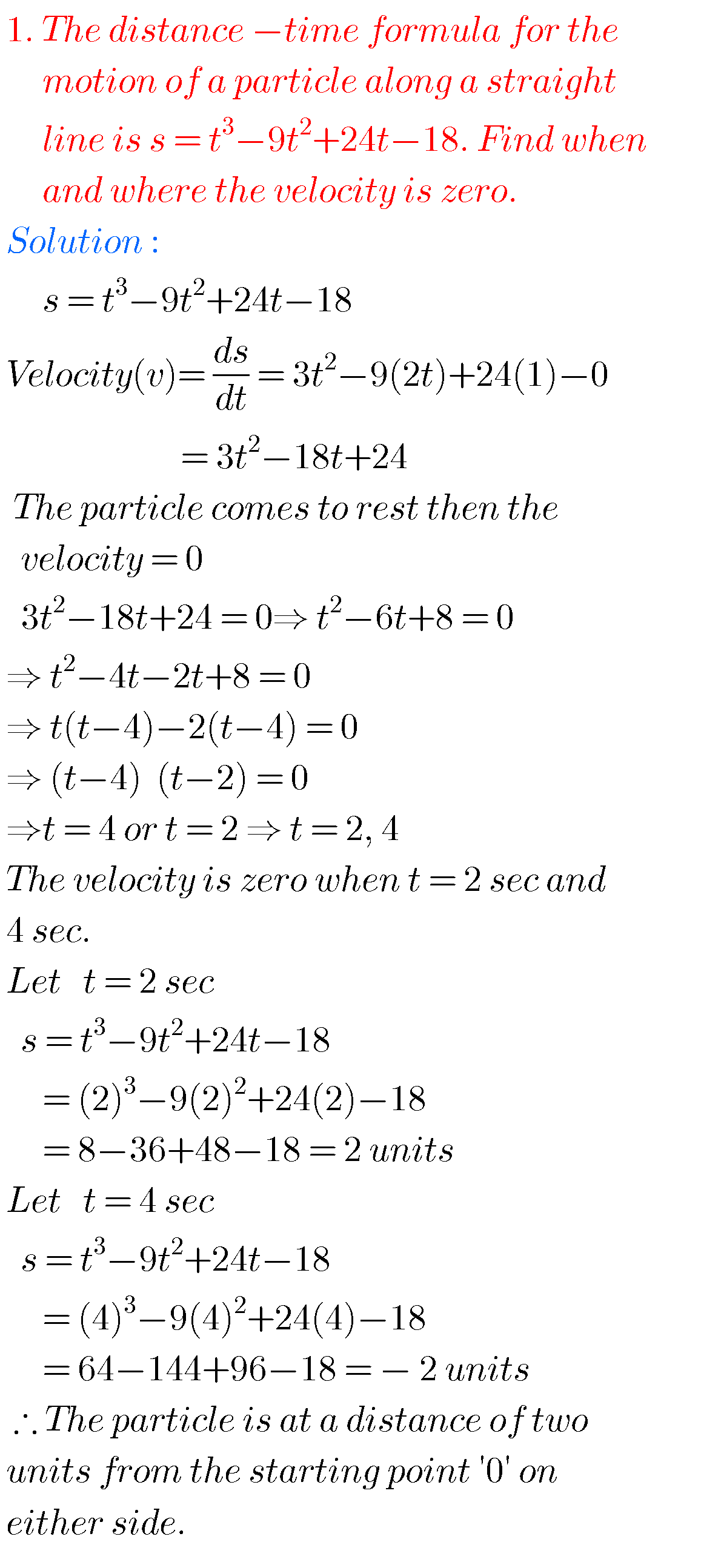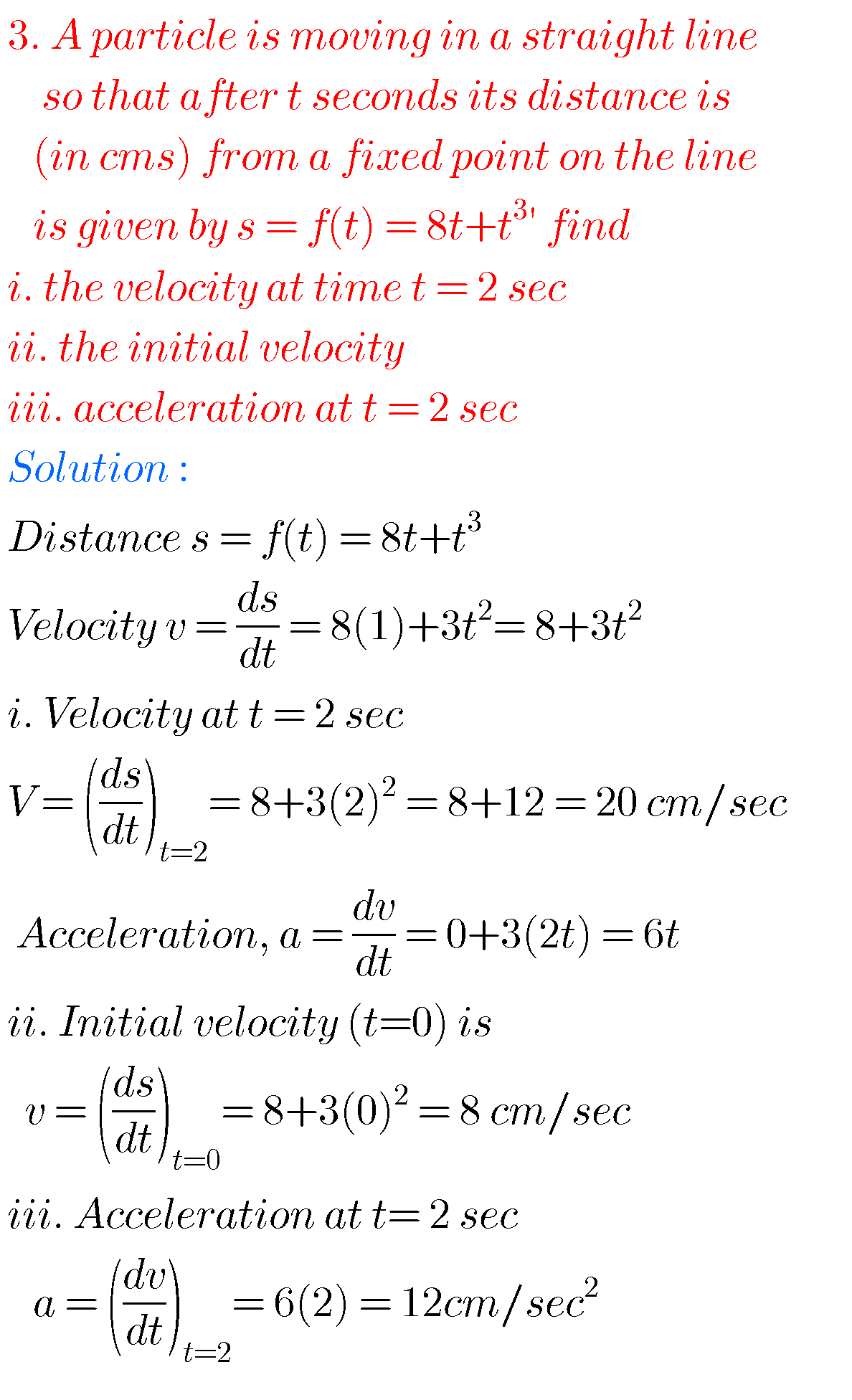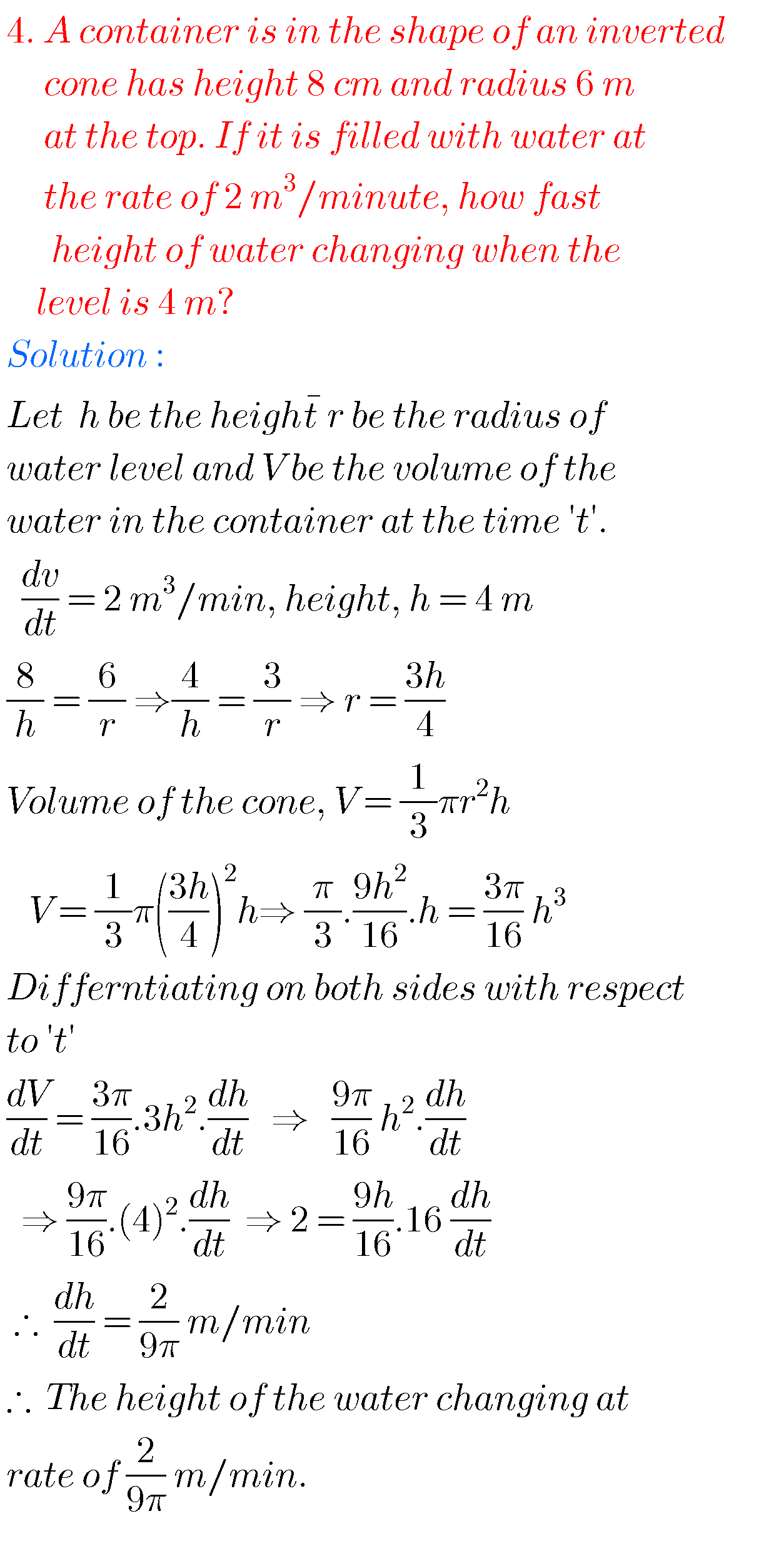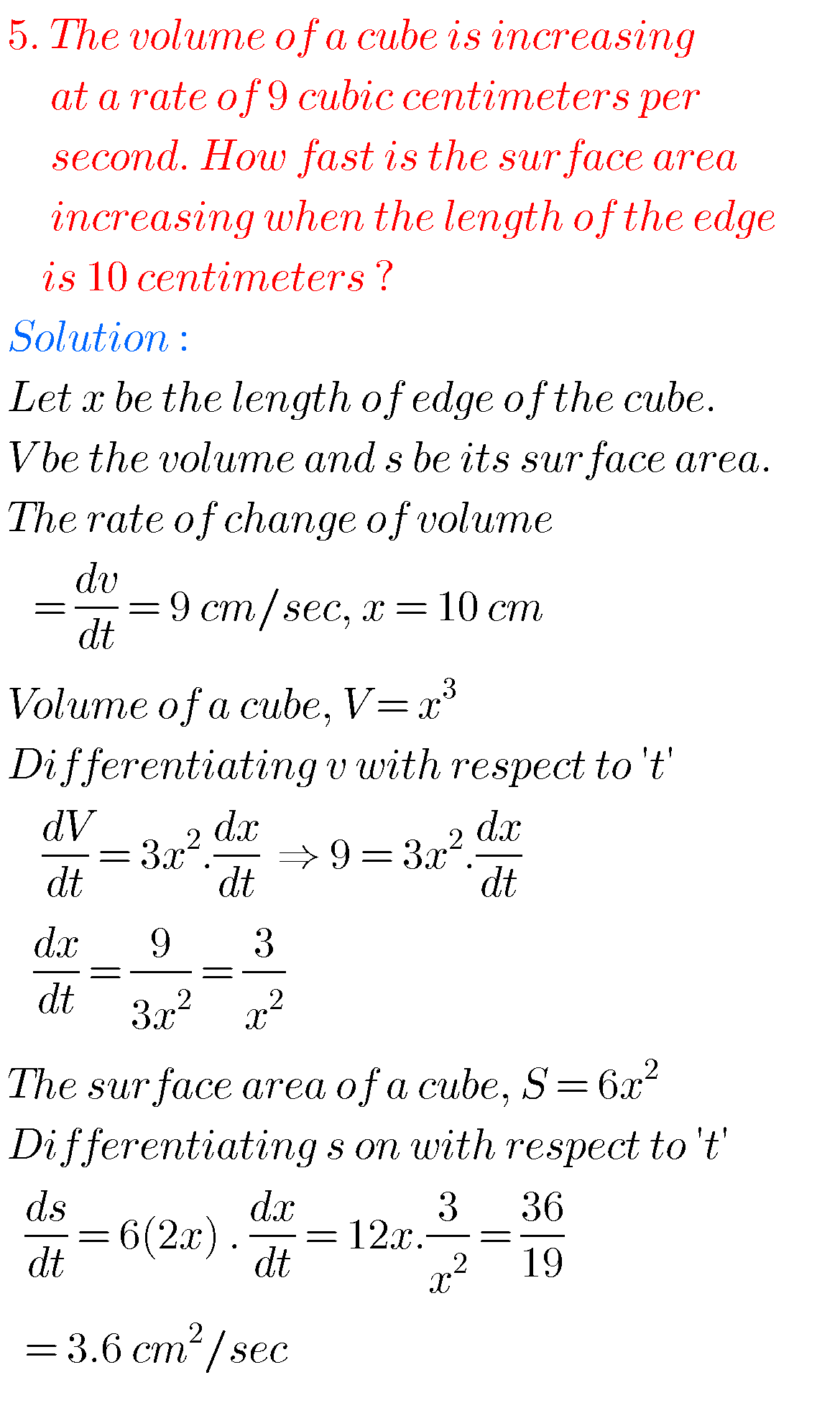# RATE MEASURE, Intermediate first year 1b chapter 10.3 problems with solutions

Mathematics intermediate first year 1A and 1B solutions for some problems. These solutions are very simple to understand.

Junior inter 1A : Functions, mathematical induction, functions, addition of vectors, trigonometric ratios upto transformations, trigonometric equations, hyperbolic functions, inverse trigonometric functions and properties of triangles.

Junior inter 1B : Locus, transformation of axes and three dimensional coordinates, straight lines, pair of straight lines, directions cosines & ratios, the planes and applications of derivatives.

You can see the solutions for junior inter maths 1b

1. Locus

The straight line sa

Straight lines la

12. Rate measure

You can also see the solutions for junior inter maths 1A

3. Matrices

## Solutions for rate measure inter maths 1bNote : Observe the solutions and try them in your own methods.

Maths real numbers solutions class 10